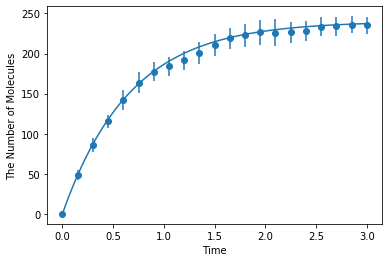# Birth-Death¶

This is for an integrated test of E-Cell4. Here, we test a simple birth-death process in volume.

In :
%matplotlib inline
from ecell4.prelude import *


Parameters are given as follows. D and radius mean a diffusion constant and a radius of molecules, respectively. Dimensions of length and time are assumed to be micro-meter and second.

In :
D = 1  # 0.01

In :
N = 20  # a number of samples

In :
y0 = {} # {'A': 60}
duration = 3
V = 8


Make a model for all algorithms. No birth reaction with more than one product is accepted.

In :
with species_attributes():

with reaction_rules():
~A > A | 45.0
A > ~A | 1.5

m = get_model()


Save a result with ode as obs, and plot it:

In :
ret1 = run_simulation(duration, y0=y0, volume=V, model=m)
ret1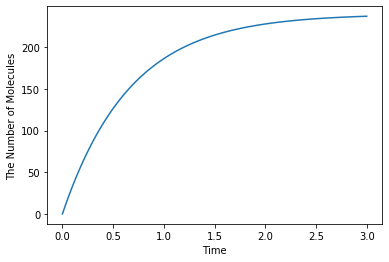Simulating with gillespie (Bars represent standard error of the mean):

In :
ret2 = ensemble_simulations(duration, ndiv=20, y0=y0, volume=V, model=m, solver='gillespie', repeat=N)
ret2.plot('o', ret1, '-')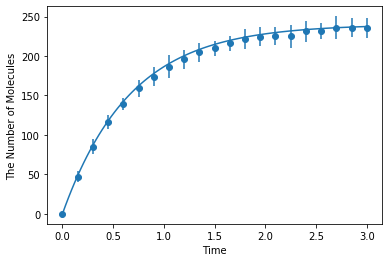Simulating with meso:

In :
ret2 = ensemble_simulations(
duration, ndiv=20, y0=y0, volume=V, model=m, solver=('meso', Integer3(3, 3, 3), 0.25), repeat=N)
ret2.plot('o', ret1, '-')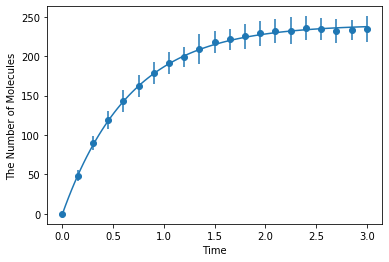Simulating with spatiocyte:

In :
ret2 = ensemble_simulations(
duration, ndiv=20, y0=y0, volume=V, model=m, solver=('spatiocyte', radius), repeat=N)
ret2.plot('o', ret1, '-')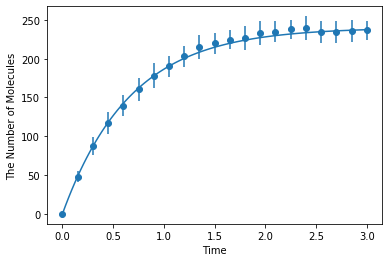Simulating with egfrd:

In :
ret2 = ensemble_simulations(
duration, ndiv=20, y0=y0, volume=V, model=m, solver=('egfrd', Integer3(8, 8, 8)), repeat=N)
ret2.plot('o', ret1, '-')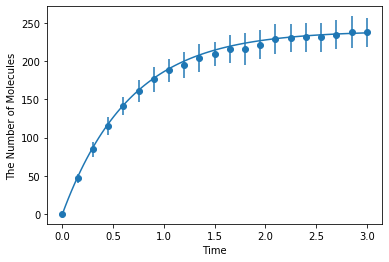Simulating with bd:

In :
ret2 = ensemble_simulations(
duration, ndiv=20, y0=y0, volume=V, model=m, solver=('bd', Integer3(8, 8, 8), 0.1), repeat=N)
ret2.plot('o', ret1, '-')